# Linear Regression: Least Squares Fit of a Straight Line to a Set of Points. Includes a Proof that the Total Variance can be Divided into Component Parts, and also Includes a Derivation of the Correlation Coefficient, Explaining its Meaning

We want to fit a straight line of the form: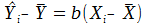(Eq. 1) or, alternatively,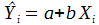(Eq. 2) to a set of data points.

Using the least squares method, we want to minimize the function: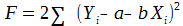(Eq. 3).

Taking partial derivatives and setting them to zero, we get: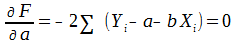(Eq. 4).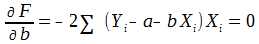(Eq. 5).

Solving these equations, we get: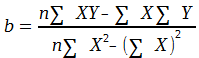(Eq. 6), and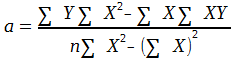(Eq. 7),

or,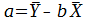(Eq. 8).

In the following, we will assume that the number of points, n, in our data set is
quite large, say, >= 100.
So to calculate variances we will simply divide sums
by n (rather than n-1 or n-2 to take into account degrees of freedom).
Consequently, the variances we will calculate are only close approximations.

Using the values of a and b that we calculated above to minimize the F function,
we have:So,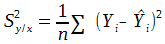(Eq. 9),

where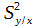is the part of the total variance,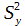that is left unexplained by the regression.

The other part of the total variance is variance explained by the regression,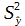The explained and unexplained variances should add up to the total variance, i.e., that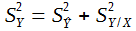(Eq. 10), and we will now prove that.

Equation 10 can also be written as: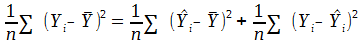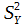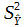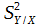We start the proof with: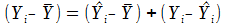(identity).

Squaring both sides and summing, we get: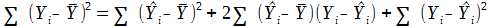.

So the theorem is true if: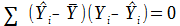.

Or equivalently if: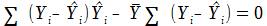(Eq. 11).

(A)                       (B)

From Equation 4, since we are using the optimum values for a and b, we know that: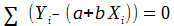, and, substituting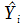for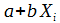, that means that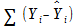is zero. So (B) above is true.
From equation 5, we know that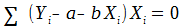. Substituting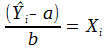, we get: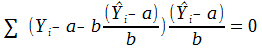Simplifying, we get: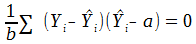,

and: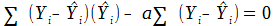. Since (B) , above, is zero, that means that (D) is zero,

(C)                            (D)

which in turn means that (C) is zero. And (C) is the same as (A), so (A) is zero.
Then, because (A) and (B) are both zero, equation 11 is zero. This proves
equation 10, that: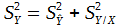, i.e., that the total variance can be separated into two
non-overlapping components, the variance explained and the variance
unexplained by the regression, that added together are equal to the total variance.

Now we will look at the relationship between the total variance and the unexplained variance and define a correlation coefficient, r.

Squaring equation 1,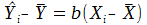, we get: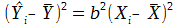.

Summing over n yields: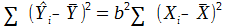, which means that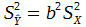.

Substituting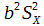forin Equation 10, we have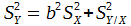, or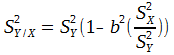A standard equation for the variance of a sample is: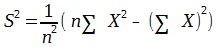.

And since, from equation 6,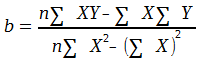, then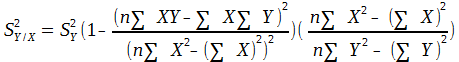, or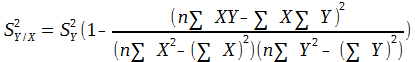The rightmost term on the right hand side of this equation is the square of the correlation coefficient, r.

So,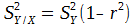(Eq. 12).

From equation 10, we know that, or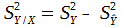.

Substituting into equation 12,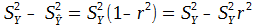.

So,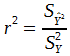In words,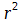is the ratio of the variance explained by the regression of Y on X to the total variance. This, in my opinion provides a better definition of the correlation coefficient than its usual definition as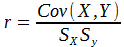.

# Cow Grazing in a Circular Pasture Problem: Solution by Calculus

```"A cow is attached by a rope to a point on the perimeter
of a circular field. How long should the rope be in terms
of the radius of the field so that the cow can graze in
exactly half of the field?"

This problem has been around in one form or another
for a long time.  You can find many solutions to it
on on the internet.  But all of the solutions I've seen
are geometric solutions, using plane geometry.  They
all chop up the pasture into various shapes and add up
the area of each one.

I have found a solution using calculus that I think is
a lot simpler and more elegant.  This solution defines
a differential of area that is centered around the point
on the perimeter of the pasture where one end of the
rope is tied.  Then it integrates from the origin (zero)
until the rope is long enough to allow the cow to graze
on half the area of the circular pasture.
Here's the derivation:

Let R be the radius of the cow pasture.
Let r be the length of the rope.
Let Θ be the angle between a line from the point where
the rope is tied on the perimeter of the pasture to the
center of the pasture, and a line of length r from where
the rope is tied on the perimeter to the edge of the
pasture.  See diagram: ``````1. BC is a circular arc of radius r centered at point A.
The length L of BC is 2Θr.
2. Cos(Θ) = AB/AD = r/(2R), so Θ = cos-1(r/(2R)).
(Note: Angle ABD is a right angle because it intercepts
a 180 degree arc.)
3. So L = 2Θr = 2r * cos-1(r/(2R)).
4. Consequently, one form of a differential of area of
the pasture is dArea = 2r * cos-1(r/(2R))dr.

We want to integrate this differential along AD starting
at point A until r is long enough so that half the area
of the pasture is enclosed, so we need to solve for x: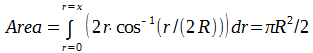Wolfram’s online integrator, integrals.wolfram.com, easily solves
the integral portion of this equation if you substitute K for R
in the integral.  Then you can let R = 1 and use WolframAlpha to
solve for the ratio of r to R that will result in half of the
area of the pasture.  The answer, to 4 decimal places is
r = R * 1.1587
```

# Accelerating Expansion of the Universe Yes or No?

Accelerating Expansion of the Universe Yes or No?

This essay contains a lot of graphs and mathematical equations that this page won't easily accommodate, so here's a link to it:

# Derivation of the Formula for Mortgage Payments

Derivation of the Formula for Mortgage Payments

A while ago, I wanted to know the reasoning behind the amount of my payments on my home mortgage. I looked in some accounting books, and all they gave was the formula. None of them gave the rationale behind it. So I sat down and derived it myself. It's not too hard.

Say we borrow an amount "A" at an interest rate of "r" per payment period. (If the payments are made monthly, "r" is the annual interest rate quoted by the bank divided by 12.) We pay back the loan in "N" payments or periods.

For example, for a 30 year mortgage on which payments are made monthly, N would be 30×12 or 360. After N payments, each of the amount "P", the loan is paid off and the amount we owe is reduced to zero.

Derivation of the Formula
The Initial amount we owe is A. At the end of the first payment period, the amount we owe has increased by rA, one payment period of interest, and we make a payment, P. So the total amount we owe after one period is
: A + rA – P, or A(1 + r) – P. We note that this A(1 + r) – P is not only an amount, but also an operator; that is, given the amount of principal outstanding at the beginning of any period, we can apply it to determine the amount of principal remaining at the end of the period.

So applying the operator A(1 + r) – P to the amount A(1 + r) – P remaining at the end of the first period, we get (A(1 + r) – P)(1 + r) – P as the amount remaining at the end of the second period. Similarly, at the end of the third period, the amount of principal remaining is: ((A(1 + r) – P)(1 + r) – P)(1 + r) – P. After N periods (applying the operator and then expanding), the amount remaining will be:

A(1 + r)^N – P( (1 + r)^(N-1) + (1 + r)^(N-2) + (1 + r)^(N-3) + … + 1 ) = 0  [Equation 1].

It equals zero, because after N periods the loan is paid off. Considering just the (1 + r)^(N-1) + (1 + r)^(N-2) + (1 + r)^(N-3) + … + 1 portion, we can reverse the order of its terms and rewrite it as: 1 + (1 + r) + (1 + r)^2 + … + (1 + r)^(N-1)

Representing this series by "S", and letting "R" equal (1 + r), we get:
S = 1 + R + R^2 + … + R^(N-1)
So, RS = R + R^2 + … + R^(N-1) + R^N
Subtracting: S – RS = 1 – R^N, and so S = (1 – R^N) / (1 – R)
Inserting this value for S back into Equation 1:

A(1 + r)^N – P( (1 – R^N) / (1 – R) ) = 0

Finally, Solving for P, the amount we pay each period, we get:

P = rA / (1 – (1 + r)^(-N))

I checked this formula against my own mortgage amount and payments and it agreed exactly! Voila! For example, for a 30 year mortgage for \$300,000 at an interest rate of 6% per year paid monthly, the parameters are: A = 300000 (the mortgage amount) r = 0.06 / 12 = 0.005 (the monthly interest rate) N = 30 x 12 = 360 (the number of payments) And the monthly payments would be: 0.005 x 300000/(1 – 1.005^(-360)) = 1798.65 dollars per month.

Another Way: Approximation with a Differential Equation
We can also use a differential equation to get a very close approximation of the payments on a mortgage.  A while ago I was trying to figure out how long it would take a bug walking along a stretching rubber band to get to the end.  After I solved that problem, it occurred to me that the problem of mortgage payments could be solved in a similar way.  It's a nice example of how a differential way of thinking can be used to solve a real-world problem.  Perhaps many problems in finance and economics can be solved using a differential approach.

We start by looking at how fast the mortgage is being paid off:  We now let A(t) be the amount we owe on the mortgage as a function of time.  Note that A(0) is the amount of the mortgage, the amount we borrowed.  Each month, the bank adds an interest amount of r * A to the mortgage and we make a payment of P.  Consequently, the amount we have remaining to pay on the mortgage changes by: dA/dt = r * A – P each month.

To solve this equation for A requires a little bit of mathematical gymnastics, but it's strictly cookbook.  It can be very easily solved by entering "solve (dA/dt = r * A – P)", without the quotes, into WolframAlpha at www.wolframalpha.com and clicking on the = sign.

The solution is: A(t) = P/r + C e^(rt), where C is a constant we need to evaluate. After a time T, the mortgage will be paid off, so we have: A(T) = P/r + C e^(rT) = 0. Solving for C, we get, C = -P/r e^(-rT). Replacing C in the solution, A(t) = (P/r) (1 – e^(r (t-T)). So, A(0), the amount of the mortgage (the amount we borrowed) is: A(0) = P/r (1 – e^(-rT)). And finally, solving for P we get: P = r A(0) / (1 – e^(-rT)). For A(0) = 300000 dollars, i = 0.06 / 12 = 0.005 percent per month, and T = 360 months, we get: P = 1797.05 dollars per month, very close to the amount we calculated before.  (But, of course, not quite good enough for the bank!)

# Algorithm for Generating a Random Grand Tour Sequence

Algorithm for Generating a Random Grand Tour Sequence

by Bob Day

1. Introduction
This post describes a method I came up with for generating a sequence for touring data blocks in a computer in random order.  The method assures that the tour will include the entire set of data blocks (a "grand tour") and that the set contains no internal cycles.  This problem occurred for me recently in conjunction with a program I wrote to determine the access latency of reads of random data blocks of memory in an array (see Section 2, below).  Due to the requirements of the program, the generation of the random block sequence had to be done in place, with no additional array storage.  No doubt this method has been discovered many times by many others, but I hadn't seen it (maybe I don't do enough reading), and I thought it was interesting.  Outside of algorithms that have their roots in mathematical theory, such as RSA encryption, it's one of the very few algorithms I've encountered in programming that wasn't intuitively obvious (at least to me) and required a mathematical proof.

2. Description of the Algorithm
a)  Allocate an array of N structures, "blocks", each of which is at least long enough to contain an index number.  Number the positions of the blocks in the array from 0 to N-1.  Think of the blocks as going in a row, from left to right.

b)  In each block, store its own position (index).  Thus block 0 will contain 0 (in addition to any other data), block 1 will contain 1, etc.

c)  Proceed block by block through the array for N-1 iterations of a loop, starting with block 0: At each block, select at random any of the blocks to its right, and swap the indexes that are stored in the blocks.  For example, at the 101st iteration, if block 100 contained 37 (due to an earlier swap), and the random block selected was 581, which still contained 581, their indexes would now be swapped so that block 100 would contain 581, and block 581, 37.

3. Proof
After I wrote the code for the above algorithm, I thought, "Well, yeah, but how do we know that they're no internal cycles?  Maybe, for example, something like this could happen: block 51 goes to block 11; block 11 goes to block 23, and block 23 goes back to block 51."  As it turns out, there can be no internal cycles.  The method generates a single "grand tour" that includes all of the blocks.  Here's the proof (it's not too hard, but it took me a while to think of it):

1.  After step a) in the preceding section, there are N cycles in the array — each block points to itself.

2.  The first iteration in step c) in the preceding section unites two blocks, block 0, and the random block chosen, into a single cycle.  There are now N-1 cycles in the array, and each of the N-1 blocks to the right of block 0 is in a different cycle. There are N-2 swaps remaining to be performed.

3.  In the second iteration in step c), block 1 and a random block to the right of block 1 are selected to have their indexes swapped.  These blocks are in different cycles (since all of the blocks to the right of block 0 are in different cycles), so swapping their indexes will unite two cycles into one.  There are now N-2 cycles in the array, and each of the N-2 blocks to the right of block 1 is in a different cycle. There are N-3 swaps remaining to be performed.

4.  With each iteration in step c) two blocks are selected that are in different cycles and their indexes are swapped, uniting two cycles into one, and reducing the number of cycles by one.  After iteration i (numbering i from 0), there are (N-i-1) cycles in the array; each of the (N-i-1) blocks to the right of the block at position i is in a different cycle, and there are (n-i-2) iterations remaining.­ All of the blocks to the right of the one we just swapped (that is, the one at position i) are all in different cycles.

5.  After the last iteration (where i equals N-2), there are (N-(N-2)-1) cycles in the array — which equals exactly one!  Voila!

2. Example Code

Here is the code I referred to in the Introduction that implements the algorithm.

//
// MemLatencyRand – A program to compute random access memory
// latency and block read times.
//
// Author: Bob Day
//
// Version 1.0
//
//
// This program may be used and distributed, with
// acknowledgment to the author, under the terms
// of the GNU General Public License.
//
#include <stdio.h>
#include <stdlib.h>
#include <string.h>
#include <sys\timeb.h>
#include <time.h>
#include <windows.h>
#include <winuser.h>
//
// Function Declarations
//
long BlockReads (long** ppMemBufL, char* pBlockBuf, long blockSizeB,
long numIterations, double* pdTime);
double Drand (long*, unsigned long*);
long Latency (long** ppMemBufL, char* pBlockBuf, long blockSizeB,
long numIterations, double* pdTime);
long NullLoop (long numIterations, double* pdTime);
unsigned long ran32 (void);
//
// Globals
//
long drand_param = -71268367;       // Initialize the parameter for Drand.
//
// Functions
//int main (int argc, char* argv[])
{
double  averageTime;
double  numIterationsD;
double  latencyTime;
double  nullLoopTime;
char*   pBlockBuf = NULL;
long**  ppMemBufL = NULL;
long**  ppMemBuf1L = NULL;
char*   pMemBufB = NULL;
long*   pTempL = NULL;
long**  ppTemp1L = NULL;
long**  ppTemp2L = NULL;
long    blockArraySizeB;
long    blockSizeB;
long    idx;
long    idxMax;
long    jdx;
long    memBufSizeB;
long    memBufSizeL;
long    memBufSizeMB;
long    numBlocks;
long    numIterations;
long    randIdx;
long    tempLong;
long    rc;

rc = 0;                                 // The default is success.
if (argc != 4
{
printf ("%s%s%s%s%s%s%s%s%s%s%s%s%s%s%s%s%s%s%s%s%s%s",
"memLatencyRand – A program to compute random access\n",
"memory latency and block read times.\n",
"Author: Bob Day\n",
"Version 1.0\n\n",
"The inputs are a block size (blockSize) in bytes, a memory buffer\n",
"size (bufSize) in megabytes, and a number of iterations (numIter).\n",
"The program allocates an array of however many blocks, each of\n",
"blockSize bytes, that it takes to fill the memory buffer.  Then\n",
"the program determines the memory latency by looping numIter times\n",
"selecting blocks at random and reading the first (4 byte) longword\n",
"in each block.  From the time required to execute this loop, and\n",
"latency time in nanoseconds.  Then the program performs a similar\n",
"loop, but this time reading entire blocks each time, and from the\n",
"timing information it gathers, computes the average read time for\n",
"a randomly selected block.\n\n",
"Call format:\n",
"       memLatencyRand blockSize bufSize numIter\n",
"Where:\n",
"       blockSize is the block size in bytes,\n",
"       bufSize is the buffer size in megabytes, and\n",
"       numIter is the number of iterations to be performed,\n");

goto exit;
}

// Get the arguments…
blockSizeB = atol(argv);             // Block size in bytes.
memBufSizeMB = atol(argv);
memBufSizeB = memBufSizeMB * 1000000;   // Memory buffer size in bytes.
numIterations = atol(argv);          // Number of iterations.
if (blockSizeB < 4)
{
printf ("%s\n", "The block size must be at least 4 bytes; exiting.");
goto exit;
}

if (blockSizeB > memBufSizeB)
{
printf ("%s\n", "The buffer size must at least the block size; exiting.");
goto exit;
}

printf ("%s%d%s\n%s%d%s\n%s%d%s\n\n",
"Block size: ", blockSizeB, " bytes.",
"Buffer size: ", memBufSizeMB, " megabytes.",
"Number of iterations: ", numIterations, ".");

// Initialize the pseudorandom number
// generator…
drand_param = time (0);                 // Generate a random number seed.
if (drand_param > 0)                    // If it's greater than zero,
drand_param = -drand_param;           // make it negative.
else if (drand_param == 0)              // If it's zero,
drand_param = -71268367;              // pick a negative number (anything).

memBufSizeL = memBufSizeB >> 2;         // Memory buffer size in longwords.
numBlocks = memBufSizeB / blockSizeB;   // The number of blocks.
blockArraySizeB = numBlocks * blockSizeB;// The total size of the array of blocks.
ppMemBufL = (long**)malloc ((blockArraySizeB + 3) & ~3); // Interpret the buffer as longs. // (Make sure the buffer is an integral number of longwords.)

if (ppMemBufL == NULL)
{
printf ("%s\n", "Error allocating main buffer; exiting.");
goto exit;
}

pBlockBuf = (char*)malloc (blockSizeB); // Allocate a buffer to do the RAM reads into.
if (pBlockBuf == NULL)
{
printf ("%s\n", "Error allocating block buffer; exiting.");
goto exit;
}

pMemBufB = (char*)ppMemBufL;            // We'll use this later.
// Initialize the buffer…
tempLong = 0;
for (idx = 0; idx < numBlocks; ++idx)   // 1. At the beginning of each block,
{                                     //    write its index.
pTempL = (long*)(pMemBufB + tempLong);
*pTempL = idx;
tempLong += blockSizeB;
}

idxMax = numBlocks – 1;
for (idx = 0, jdx = 1; idx < idxMax; ++idx, ++jdx)
{                                     // 2. Shuffle the indexes…
// (Note: This method of shuffling produces a single loop that includes all of the blocks.)
randIdx = (long)((double)(numBlocks – jdx) * Drand (&drand_param, NULL));

// Pick a number in the range [0, numBlocks-jdx-1].
// Swap it with the current item:
ppTemp1L = (long**)(pMemBufB + idx * blockSizeB);
ppTemp2L = (long**)(pMemBufB + (randIdx + jdx) * blockSizeB);
pTempL = *ppTemp1L;
*ppTemp1L = *ppTemp2L;
*ppTemp2L = pTempL;
}

tempLong = 0;                           // 3. Turn the indexes into actual addresses…
for (idx = 0; idx < numBlocks; ++idx)
{
ppTemp1L = (long**)(pMemBufB + tempLong);
*ppTemp1L = (long*)(pMemBufB + (__int64)(*ppTemp1L) * blockSizeB);
tempLong += blockSizeB;
}

rc = NullLoop (numIterations, &nullLoopTime);
if (rc != 0)                            // If error…
{
printf ("%s\n", "Error computing loop overhead; exiting.");
goto exit;
}

rc = Latency (ppMemBufL, pBlockBuf, blockSizeB, numIterations, &latencyTime);
if (rc != 0)                            // If error…
{
printf ("%s\n", "Error computing latency; exiting.");
goto exit;
}

numIterationsD = (double)numIterations;
averageTime = ((latencyTime – nullLoopTime) / numIterationsD) * 1e9;
printf ("%s%s%f%s\n\n",
"(single 4 byte integer read per block): ",
averageTime,
" nanoseconds.");

if (rc != 0)                            // If error…
{
printf ("%s\n", "Error doing block reads; exiting.");
goto exit;
}

averageTime = ((blockReadTime – nullLoopTime) / numIterationsD) * 1e9;
printf ("%s%f%s\n", "Random block read time: ", averageTime, " nanoseconds.");

exit:
if (ppMemBufL != NULL) free (ppMemBufL);
if (pBlockBuf != NULL) free (pBlockBuf);
printf ("%s", "\n");
// printf ("%s", "\nPress any key to exit:");
// getchar();
// Sleep (INFINITE);

if (rc != 0) rc = 0;
return rc;
}

long Latency (long** ppMemBufL, char* pBlockBuf, long blockSizeB,
long numIterations, double* pdTime)
{
double  dTime;
HANDLE  hProcess = NULL;
long    idx;
long    rc;
long    t1;
long    t2;
rc = 0;                                 // The default is success.
*pdTime = (double)0.0;
hProcess = GetCurrentProcess ();
rc = SetPriorityClass (hProcess, REALTIME_PRIORITY_CLASS);
// rc = SetPriorityClass (hProcess, HIGH_PRIORITY_CLASS);
if (rc == 0)
{
printf ("%s\n", "Error setting priority class.");
rc = -1;
goto exit;
}

if (rc == 0)
{
printf ("%s\n", "Error setting thread priority.");
rc = -1;
goto exit;
}

t1 = timeGetTime();                     // Get the starting time in milliseconds.
for (idx = 0; idx < numIterations; ++idx)
{
ppMemBufL = (long**)(*ppMemBufL);
// memcpy (pBlockBuf, (char*)ppMemBufL + 4, blockSizeB – 4);
}
t2 = timeGetTime();                     // Get the ending time in milliseconds.
rc = SetPriorityClass (hProcess, NORMAL_PRIORITY_CLASS);
if (rc == 0)
{
printf ("%s\n", "Error setting priority class.");
rc = -1;
goto exit;
}

if (rc == 0)
{
printf ("%s\n", "Error setting thread priority.");
rc = -1;
goto exit;
}

if ((long)(__int64)ppMemBufL == NULL)   // Do Something with ppMemBufL to make
{                                     // sure the compiler won't optimize it
rc = -1;                              // out.  (If ppMemBufL is NULL, it's
goto exit;                            // definitely an error!)
}

dTime = ((double)(t2 – t1)) / (double)1000.0;
// printf ("Latency time (including loop overhead): %f seconds.\n", dTime);
// Display the time in seconds.
*pdTime = dTime;                        // Return the time.
rc = 0;                                 // If we get here, we were successful.
exit:
return rc;
} // End of Latency

long NullLoop (long numIterations, double* pdTime)
{
double  dTime;
HANDLE  hProcess = NULL;
long    rc;
long    t1;
long    t2;
rc = 0;                                 // The default is success.
*pdTime = (double)0.0;
hProcess = GetCurrentProcess ();
rc = SetPriorityClass (hProcess, REALTIME_PRIORITY_CLASS);
// rc = SetPriorityClass (hProcess, HIGH_PRIORITY_CLASS);
if (rc == 0)
{
printf ("%s\n", "Error setting priority class.");
rc = -1;
goto exit;
}

if (rc == 0)
{
printf ("%s\n", "Error setting thread priority.");
rc = -1;
goto exit;
}

t1 = timeGetTime();                     // Get the starting time in
// milliseconds.
__asm
{
push    eax
mov     eax, numIterations
test    eax, eax
jle L2
L1:   dec     eax
jne L1
L2:   pop     eax
}

t2 = timeGetTime();                     // Get the ending time in milliseconds.
rc = SetPriorityClass (hProcess, NORMAL_PRIORITY_CLASS);
if (rc == 0)
{
printf ("%s\n", "Error setting priority class.");
rc = -1;
goto exit;
}

if (rc == 0)
{
printf ("%s\n", "Error setting thread priority.");
rc = -1;
goto exit;
}

dTime = ((double)(t2 – t1)) / (double)1000.0;
// printf ("Loop overhead time = %f seconds.\n", dTime);
// Display the time in seconds.
*pdTime = dTime;                        // Return the time.
rc = 0;                                 // If we get here, we were successful.
exit:
return rc;
} // End of NullLoop.

long BlockReads (long** ppMemBufL, char* pBlockBuf, long blockSizeB,
long numIterations, double* pdTime)
{
double  dTime;
HANDLE  hProcess = NULL;
long    idx;
long    rc;
long    t1;
long    t2;

rc = 0;                                 // The default is success.
*pdTime = (double)0.0;
hProcess = GetCurrentProcess ();
rc = SetPriorityClass (hProcess, REALTIME_PRIORITY_CLASS);
// rc = SetPriorityClass (hProcess, HIGH_PRIORITY_CLASS);
if (rc == 0)
{
printf ("%s\n", "Error setting priority class.");
rc = -1;
goto exit;
}

if (rc == 0)
{
printf ("%s\n", "Error setting thread priority.");
rc = -1;
goto exit;
}

t1 = timeGetTime();                     // Get the starting time in milliseconds.
for (idx = 0; idx < numIterations; ++idx)
{
ppMemBufL = (long**)(*ppMemBufL);
memcpy (pBlockBuf, (char*)ppMemBufL + 4, blockSizeB – 4);
}

t2 = timeGetTime();                     // Get the ending time in milliseconds.
rc = SetPriorityClass (hProcess, NORMAL_PRIORITY_CLASS);
if (rc == 0)
{
printf ("%s\n", "Error setting priority class.");
rc = -1;
goto exit;
}

if (rc == 0)
{
printf ("%s\n", "Error setting thread priority.");
rc = -1;
goto exit;
}

if ((long)(__int64)ppMemBufL == NULL)   // Do Something with ppMemBufL to make
{                                     // sure the compiler won't optimize it
rc = -1;                              // out.  (If ppMemBufL is NULL, it's
goto exit;                            // definitely an error!)
}

dTime = ((double)(t2 – t1)) / (double)1000.0;
// Display the time in seconds.
*pdTime = dTime;                        // Return the time.
rc = 0;                                 // If we get here, we were successful.

exit:
return rc;

/******************************************************************************/

/*                                                                            */

/* FUNCTION: ran32                                                            */
/*                                                                            */
/* DESCRIPTION:                                                               */
/*   Returns a 32 bit pseudorandom number.                                    */
/*                                                                            */
/******************************************************************************/
unsigned long ran32 (void)
{
unsigned long   rand1;
unsigned long   rand2;
Drand (&drand_param, &rand1);       /* Get a 30 bit random number, and        */
Drand (&drand_param, &rand2);       /* another one.                           */
return ((rand1 & 0x00FFFF00) << 8) | ((rand2 & 0x00FFFF00) >> 8);
}
/******************************************************************************/
/*                                                                            */
/* End of ran32                                                               */
/*                                                                            */
/******************************************************************************/

/******************************************************************************/
/*                                                                            */
/* FUNCTION: Drand                                                            */
/*                                                                            */
/* DESCRIPTION:                                                               */
/*   Long period random number generator of L'Ecuyer with Bays-Durham shuffle */
/*   and added safeguards.  Returns a 31 bit uniform random value.  Call with */
/*   idum a negative integer to initialize; thereafter, do not alter idum     */
/*   between successive deviates in a sequence.                               */
/*                                                                            */
/* SOURCE:                                                                    */
/*   Numerical Recipes in C, Second Edition, 1992                             */
/*                                                                            */
/******************************************************************************/
/*                                                                            */
/* Symbol Definitions                                                         */
/*                                                                            */
#define IM1 2147483563
#define IM2 2147483399
#define AM  (1.0/IM1)
#define IMM1    (IM1-1)
#define IA1 40014
#define IA2 40692
#define IQ1 53668
#define IQ2 52774
#define IR1 12211
#define IR2 3791
#define NTAB    32
#define NDIV    (1+IMM1/NTAB)
/*                                                                            */
/* Start of Code                                                              */
/*                                                                            */
double Drand (long *idum, unsigned long* pIrand)
{
double      randNum;
static long idum2 = 123456789;
static long iy = 0;
static long iv[NTAB];
long        j;
long        k;

if (*idum <= 0)                         /* If we're initializing…           */
{
*idum = (*idum == 0) ? 1 : -(*idum);  /* (Be sure to prevent idum == 0).    */
idum2 = *idum;
for (j = NTAB+7; j >= 0; –j)         /* Load the shuffle table (after 8    */
{                                   /* warm-ups).                         */
k = (*idum)/IQ1;
*idum = IA1 * (*idum – k*IQ1) – k*IR1;
if (*idum < 0) *idum += IM1;
if (j < NTAB) iv[j] = *idum;
}

iy = iv;
}

k = (*idum)/IQ1;
*idum = IA1 * (*idum – k*IQ1) – k*IR1;  /* Compute idum = (IA1 * idum) % IM1  */
if (*idum < 0) *idum += IM1;            /* without overflows by Schrage's     */
/* Method.                            */
k = idum2/IQ2;
idum2 = IA2 * (idum2 – k*IQ2) – k*IR2;  /* Compute idum2 = (IA2 * idum) % IM2 */
if (idum2 < 0) idum2 += IM2;            /* likewise.                          */
j = iy/NDIV;                            /* Will be in the range 0..NTAB-1.    */
iy = iv[j] – idum2;                     /* Here idum is shuffled; idum and    */
iv[j] = *idum;                          /* idum2 are combined to generate     */
/* output.                            */
if (iy < 0) iy += IMM1;
if (pIrand != NULL) *pIrand = iy;
randNum = ((double)(iy & 0x1FFFFFFF))/(double)536870912.0;
if ((randNum < (double)0.0) || (randNum >= (double)1.0))
{
printf ("Value out of range in Drand function (%f).\n", randNum);
}

return randNum;                         /* Return R in range 0.0 <= R < 1.0   */
}
/******************************************************************************/
/*                                                                            */
/* End of Drand                                                               */
/*                                                                            */
/******************************************************************************/

# The Mathematics of Chipkill ECC

The Mathematics of Chipkill ECC

by Bob Day

OVERVIEW
Chipkill ECC is an advanced form of computer memory error checking and correction that corrects not only single bit errors but many multi-bit errors up to 4 bits long.  Originally, the chipkill circuitry was located on the memory module itself, which prevented the use of standard memory modules.  Later, the chipkill circuitry was placed either on the northbridge chipset of the computer’s motherboard or within the CPU.  This allowed the
use of standard SDRAM (for example DDR2 or DDR3) memory modules.

Chipkill operates on 4-bit nibbles (1/2 bytes), which, in the parlance of chipkill, are called “symbols”.  If just one symbol, i.e. nibble, is erroneous, chipkill can fully correct it — all four bits if necessary.  But if more than one symbol is erroneous, chipkill can only detect it.  As a consequence of its operating on nibbles, chipkill is most effective if used with memory modules built with x4 (4-bit width) chips because each symbol corresponds to a chip.  In that case, multi-bit errors will be isolated to a single chip or symbol because of the physical distance between the chips.  Contrary to many posts on the internet, chipkill will also work just fine with memory modules built with x8 (8-bit width) chips.  It’s less effective though with x8 chips because a multi-bit error could then straddle a nibble boundary, thus causing a double symbol error that would only be detected.

Also, contrary to several posts on the internet, chipkill has nothing whatsoever to do with registered memory.  It makes no difference whether the memory modules used with chipkill are registered or unbuffered.  Unfortunately for desktop motherboards though, x4 memory modules only seem to be available in the registered version.

MATHEMATICS
I recently surfed the Internet in at attempt to find out more about the mathematics of how Chipkill ECC works.  I didn’t discover much, but I found out that it involves Galois Field mathematics!  Omigosh!  That’s really deep, obscure, arcane, and recondite!!  Nevermind complicated!!  I could never understand that!  But actually it turns out to be really really simple.  All you need to know is the Galois multiplication table from 0 to 15!  Here it is:

Multiplicand

0  1  2  3  4  5  6  7  8  9  a  b  c  d  e  f
M’plier
0        0  0  0  0  0  0  0  0  0  0  0  0  0  0  0  0
1        0  1  2  3  4  5  6  7  8  9  a  b  c  d  e  f
2        0  2  4  6  8  a  c  e  3  1  7  5  b  9  f  d
3        0  3  6  5  c  f  a  9  b  8  d  e  7  4  1  2
4        0  4  8  c  3  7  b  f  6  2  e  a  5  1  d  9

5        0  5  a  f  7  2  d  8  e  b  4  1  9  c  3  6
6        0  6  c  a  b  d  7  1  5  3  9  f  e  8  2  4
7        0  7  e  9  f  8  1  6  d  a  3  4  2  5  c  b
8        0  8  3  b  6  e  5  d  c  4  f  7  a  2  9  1

9        0  9  1  8  2  b  3  a  4  d  5  c  6  f  7  e
a        0  a  7  d  e  4  9  3  f  5  8  2  1  b  6  c
b        0  b  5  e  a  1  f  4  7  c  2  9  d  6  8  3

c        0  c  b  7  5  9  e  2  a  6  1  d  f  3  4  8
d        0  d  9  4  1  c  8  5  2  f  b  6  3  e  a  7
e        0  e  f  1  d  3  2  c  9  7  6  8  4  a  b  5
f        0  f  d  2  9  6  4  b  1  e  c  3  8  7  5  a

That’s it!  We’ll do a little division later, but that’s just multiplication by the inverse — you find the number to multiply the divisor by so that the product is 1 and multiply by that number.  For example, dividing by 9 is the same as multiplying by 2, as you can verify from the table. When memory is organized for Chipkill, numbers are read in 128 bits at a time, along with 16 check bits, making a total of 144 bits for each number. Chipkill views each number as consisting of 32 4-bit “nibbles”, which I’ll call N0 through N31.  Chipkill also divides the 16 check bits into 4-bit nibbles, which I’ll label C0 through C3.  When the computer stores a number in memory, it calculates values for the check nibbles and stores them along with the number.  The check nibble calculation is long, but not complicated:

C0 = N0 + 2*N1 + 3*N2 + 4*N3 + 5*N4 + 6*N5 + 7*N6 + 8*N7 + 9*N8 + a*N9 + b*N10 + c*N11 + d*N12 + e*N13 + f*N14 + N15 + 2*N16 + 3*N17 + 4*N18 + 5*N19 + 6*N20 + 7*N21 + 8*N22 + 9*N23 + a*N24  + b*N25 + c*N26 + d*N27 + e*N28 + f*N29 + N31

C1 = N0 + N1 + N2 + N3 + N4 + N5 + N6 + N7 + N8 + N9  + N10 + N11 + N12 + N13 + N14 + N30 + N31

C2 = N15 + N16 + N17 + N18 + N19 + N20 + N21 + N22 + N23 + N24  + N25 + N26 + N27 + N28 + N29 + N30 + N31

C3 = N0 + 9*N1 + e*N2 + d*N3 + b*N4 + 7*N5 + 6*N6 + f*N7 + 2*N8 + c*N9 + 5*N10 + a*N11 + 4*N12 + 3*N13 + 8*N14 + N15 + 9*N16 + e*N17 + d*N18 + b*N19 + 7*N20 + 6*N21 + f*N22 + 2*N23 + c*N24  + 5*N25 + a*N26 + 4*N27 + 3*N28 + 8*N29 + N30

The ‘*’ symbols in the above equations stand for Galois Field multiplication (use the table!), and the ‘+’ signs mean XOR (exclusive or). When the computer reads a number from memory, it runs through the same calculation again on the number it has read, and produces another set of check nibbles that I’ll call C0′ through C3′ (“C0 prime” through “C3 prime”).  Then it compares the two sets of check nibbles by combining them into a set of nibbles called a “syndrome” in the terminology of Chipkill.  The syndrome nibbles are labeled S0 through S3:

S0 = C0 + C0′
S1 = C1 + C1′
S2 = C2 + C2′
S3 = C3 + C3′

Again, the ‘+’ signs mean XOR. Now the computer looks at the syndrome nibbles to see whether there’s an error.

First, notice that if there’s no error, each check nibble will be the same as its primed counterpart, causing the XOR of each check nibble with its primed counterpart to be zero.  Consequently, S0 through S3 will all be zero.

Let’s see what happens if just one of the nibbles, say N7, is read in error. That would cause S0, S1, and S3 to be nonzero, since N7 appears in each of those. How can we determine that it’s N7?  We notice that the only thing that causes a syndrome nibble to be nonzero is the check nibble that’s in error. In this example, we know that the error we’re looking for is among the first 15 nibbles, N0 through N14, since S1 is nonzero and S2 is zero.  Knowing that, we divide S0 by S1.  Looking at the equations for C0 and C1 above, we see that the result will be 8 for our example.  Since the nibbles are numbered from zero, we subtract 1, which designates N7 to be the erroneous nibble.

How should we fix it?  The current value (the value we read from memory) of N7 is that of the erroneous nibble, and S1 is the XOR of the erroneous N7 with the original correct value of N7.  So if we XOR the erroneous value we have with S1, we will recover the original correct value.

An error in the second 15 nibbles (N15 through N29) can be found and fixed in the same way.

Locating an error in nibble 30 or 31 is similar.  Say S0 is zero and S1 and S2 are nonzero and equal.  Then we know immediately that N30 is erroneous because it appears in S1 and S2 but not in S0.  The correct value is recovered by XORing the value we have for N30 with either S1 or S2.  Note that S3 will also have the same value as S1 and S2.

If just one of the syndrome nibbles is nonzero, we know that the corresponding check nibble is in error.  It can be corrected by recalculating it from the data nibbles, N0 through N31.

Now, let’s consider double error detection.  In every case, if there is an error just in a single nibble, the number of nonzero syndrome nibbles is odd, so if two or four of the syndrome nibbles are nonzero, its a double error or more. It’s also at least a double error if the following two conditions apply:

a)  S1 or S2 is zero and the rest of the syndrome nibbles are nonzero.
b)  Letting X represent whichever of S1 or S2 is nonzero, the position given by
S0/X does not equal the position given by X/S3.  Why?  Let’s pick on N7 again
and let it be erroneous.  Then, if it’s a single error, S0/X = 8*N7/N7 which
equals 8, and X/S3 should also equal 8 because X/S3 = N7/(f*N7) =

N7*(8/N7) = 8, since 8 is the Galois multiplicative inverse of ‘f’ (from the table).
If the positions are not the same, then it’s not a single error, so it must be a
double error or more.

Finally, it’s at least a double error if S1 and S2 are nonzero and the nonzero syndrome nibbles are not all equal (otherwise it would be a single nibble error in N30 or N31).